#0
0
01. 云栖社区>
2. 博客>
3. 正文

## QRCode

### 尺寸

QRCode 有不同的 Version ，不同的 Version 对应着不同的尺寸。将最小单位的黑白块称为 module ，则 QRCode 尺寸的公式如下：

Version V = ((V-1)*4 + 21) ^ 2 modules

• Version 1 : 21 * 21 modules
• Version 2 : 25 * 25 modules
• Version 40: 177 * 177 modules

### 分类

QRCode 分为 Model 1、Model 2、Micro QR 三类：

• Model 1 ：是 Model 2 和 Micro QR 的原型，有 Version 1 到 Version 14 共14种尺寸。
• Model 2 ：是 Model 1 的改良版本，添加了对齐标记，有 Version 1 到 Version 40 共40种尺寸。
• Micro QR ：只有一个定位标记，最小尺寸是 11*11 modules 。

### 组成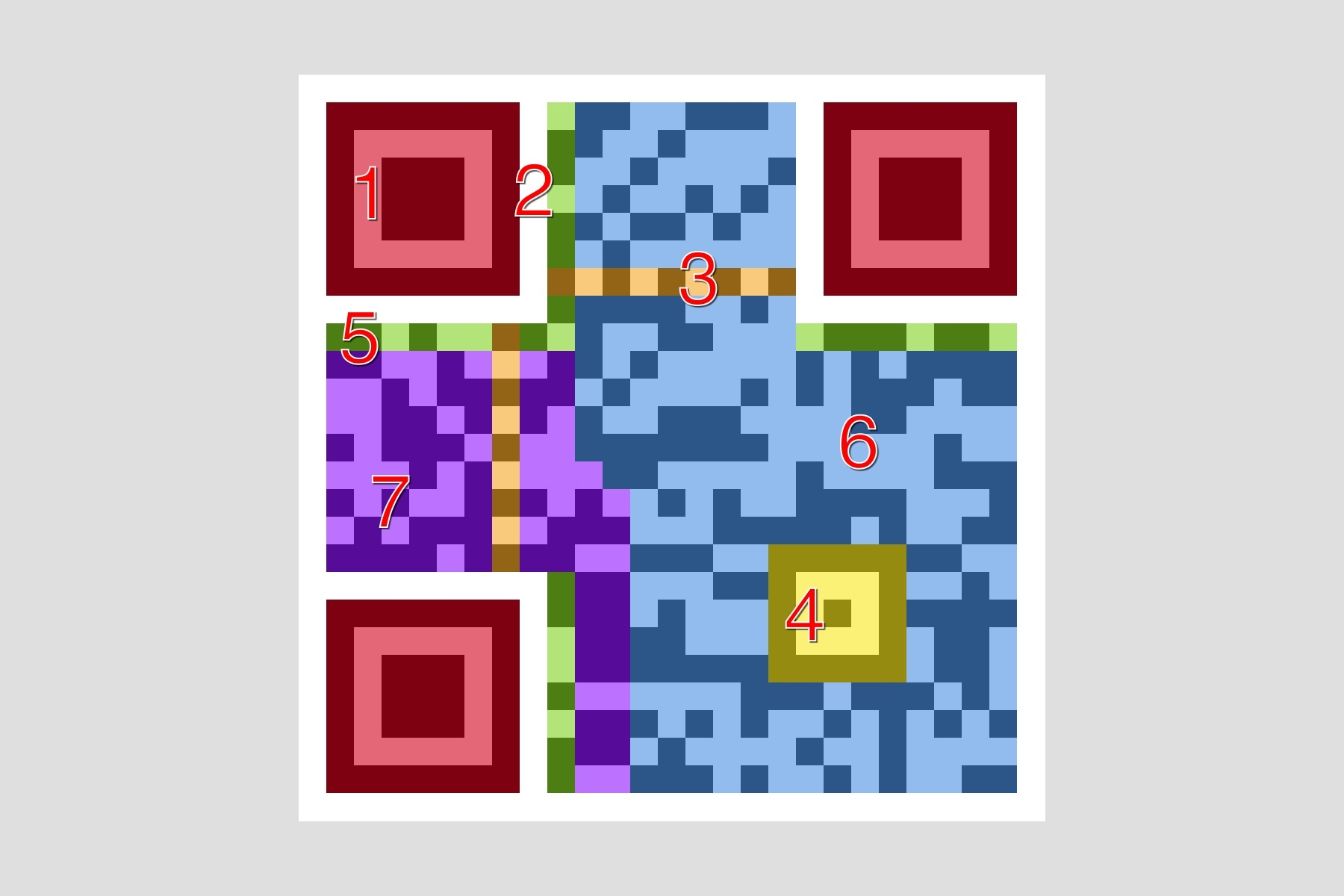QRCode 主要由以下

QRCode 主要由以下部分组成：

• 1 - Position Detection Pattern：位于三个角落，可以快速检测二维码位置。
• 2 - Separators：一个单位宽的分割线，提高二维码位置检测的效率。
• 3 - Timing Pattern：黑白相间，用于修正坐标系。
• 4 - Alignment Patterns：提高二维码在失真情况下的识别率。
• 5 - Format Information：格式信息，包含了错误修正级别和掩码图案。
• 6 - Data：真正的数据部分。
• 7 - Error Correction：用于错误修正，和 Data 部分格式相同。

## OpenCV

### contours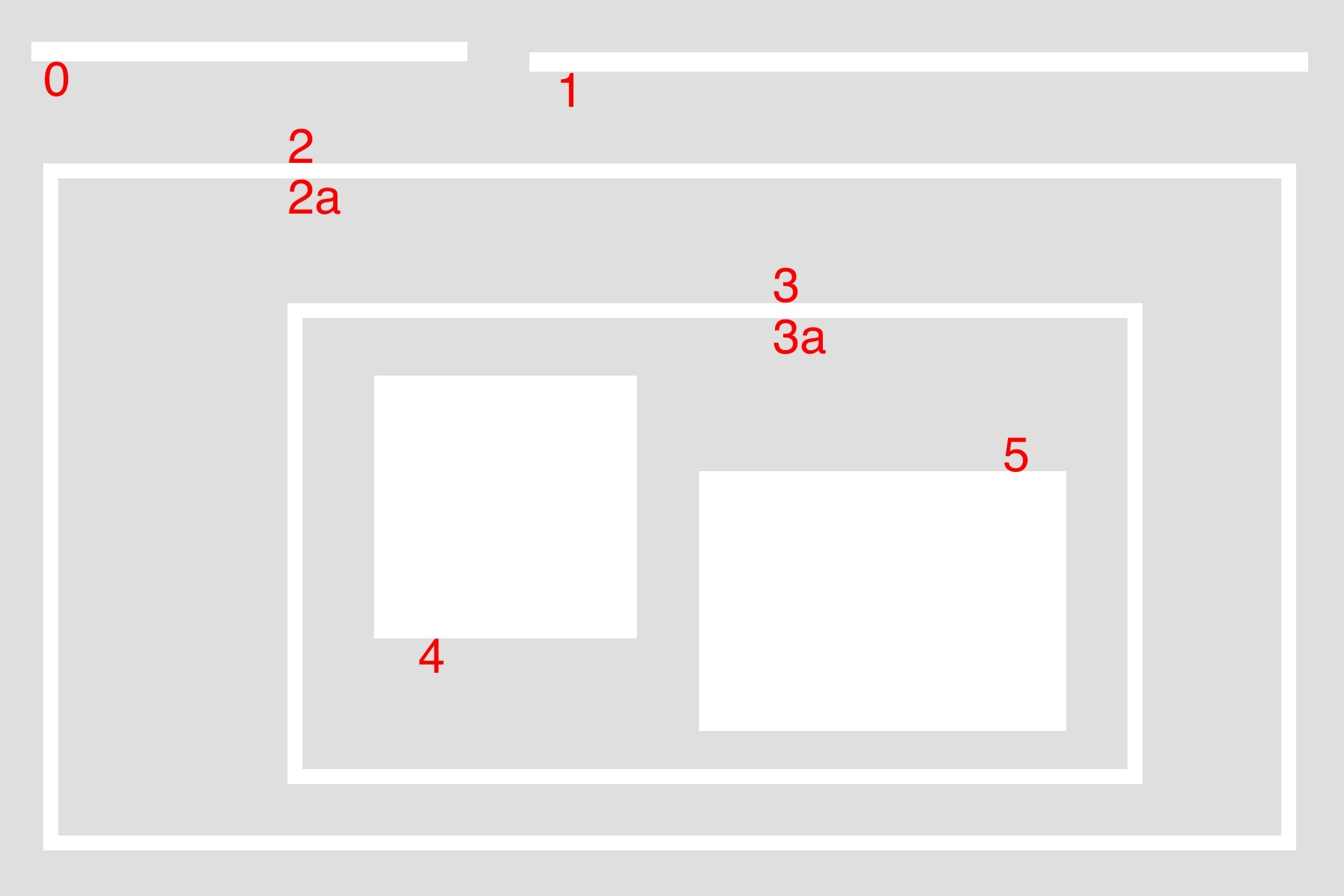• external & internal：对于最大的包围盒而言，2 是外部轮廓（external），2a 是内部轮廓（internal）。
• parent & child：2 是 2a 的父轮廓（parent），2a 是 2 的子轮廓（child），3 是 2a 的子轮廓，同理，3a 是 3 的子轮廓，4 和 5 都是 3a 的子轮廓。
• external | outermost：0、1、2 都属于最外围轮廓（outermost）。
• hierarchy level：0、1、2 是同一层级（same hierarchy），都属于 hierarchy-0 ，它们的第一层子轮廓属于 hierarchy-1 。
• first child：4 是 3a 的第一个子轮廓（first child）。实际上 5 也可以，这个看个人喜好了。

[Next, Previous, First_Child, Parent]

• Next：同一层级的下一个轮廓。在上图中， 0 的 Next 就是 1 ，1 的 Next 就是 2 ，2 的 Next 是 -1 ，表示没有下一个同级轮廓。
• Previous：同一层级的上一个轮廓。比如 5 的 Previous 是 4， 1 的 Previous 就是 0 ，0 的 Previous 是 -1 。
• First_Child：第一个子轮廓，比如 2 的 First_Child 就是 2a ，像 3a 这种有两个 Child ，只取第一个，比如选择 4 作为 First_Child 。
• Parent：父轮廓，比如 4 和 5 的 Parent 都是 3a ，3a 的 Parent 是 3 。

findContours

findContours 是寻找轮廓的函数，函数定义如下：

cv2.findContours(image, mode, method) → image, contours, hierarchy

• image：资源图片，8 bit 单通道，一般需要将普通的 BGR 图片通过 cvtColor 函数转换。
• mode：边缘检测的模式，包括：
• CV_RETR_EXTERNAL：只检索最大的外部轮廓（extreme outer），没有层级关系，只取根节点的轮廓。
• CV_RETR_LIST：检索所有轮廓，但是没有 Parent 和 Child 的层级关系，所有轮廓都是同级的。
• CV_RETR_CCOMP：检索所有轮廓，并且按照二级结构组织：外轮廓和内轮廓。以前面的大图为例，0、1、2、3、4、5 都属于第0层，2a 和 3a 都属于第1层。
• CV_RETR_TREE：检索所有轮廓，并且按照嵌套关系组织层级。以前面的大图为例，0、1、2 属于第0层，2a 属于第1层，3 属于第2层，3a 属于第3层，4、5 属于第4层。
• method：边缘近似的方法，包括：
• CV_CHAIN_APPROX_NONE：严格存储所有边缘点，即：序列中任意两个点的距离均为1。
• CV_CHAIN_APPROX_SIMPLE：压缩边缘，通过顶点绘制轮廓。

drawContours

drawContours 是绘制边缘的函数，可以传入 findContours 函数返回的轮廓结果，在目标图像上绘制轮廓。函数定义如下：

Python: cv2.drawContours(image, contours, contourIdx, color) → image

• image：目标图像，直接修改目标的像素点，实现绘制。
• contours：需要绘制的边缘数组。
• contourIdx：需要绘制的边缘索引，如果全部绘制则为 -1。
• color：绘制的颜色，为 BGR 格式的 Scalar 。
• thickness：可选，绘制的密度，即描绘轮廓时所用的画笔粗细。
• lineType: 可选，连线类型，分为以下几种：
• LINE_4：4-connected line，只有相邻的点可以连接成线，一个点有四个相邻的坑位。
• LINE_8：8-connected line，相邻的点或者斜对角相邻的点可以连接成线，一个点有四个相邻的坑位和四个斜对角相邻的坑位，所以一共有8个坑位。
• LINE_AA：antialiased line，抗锯齿连线。
• hierarchy：可选，如果需要绘制某些层级的轮廓时作为层级关系传入。
• maxLevel：可选，需要绘制的层级中的最大级别。如果为1，则只绘制最外层轮廓，如果为2，绘制最外层和第二层轮廓，以此类推。

### moments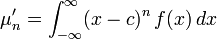• 原点矩（raw moment）：相对原点的矩，即当 c 为 0 的时候。1阶原点矩为期望，也成为中心。
• 中心矩（central moment）：相对于中心点的矩，即当 c 为 E(x) 的时候。1阶中心矩为0，2阶中心矩为方差。

raw moment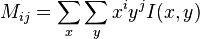• M 00 相当于权重系数为 1 。将所有 I(x, y) 相加，对于二值图像而言，相当于将每个点记为 1 然后求和，也就是图像的面积；对于灰度图像而言，则是图像的灰度值的和。
• M 10 相当于权重为 x 。对二值图像而言，相当于将所有的 x 坐标相加。
• M 01 相当于权重为 y 。对二值图像而言，相当于将所有的 y 坐标相加。
• 图像的几何中心（centroid）等于 (M 10 / M 00 , M 01 / M 00 )。

central moment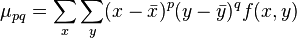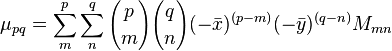• 平移不变性（translation invariants）：中心矩本身就具有平移不变性，因为它是相对于自身的中心的分布统计，相当于是采用了相对坐标系，而平移改变的是整体坐标。
• 缩放不变性（scale invariants）：为了实现缩放不变性，可以构造一个规格化的中心矩，即将中心矩除以 (1+(i+j)/2) 阶的0阶中心矩，具体公式见 《 Wiki: scale invariants 》。
• 旋转不变性（rotation invariants）：通过2阶和3阶的规格化中心矩可以构建7个不变矩组，构成的特征量具有旋转不变性。具体可以看 《 Wiki: rotation invariants 》。

Hu moment 和 Zernike moment 之类的内容就不继续展开了，感兴趣的可以翻阅相关文章。

## OpenCV + QRCode

• 加载图像，并且进行一些预处理，比如通过高斯模糊去噪。
• 通过 Canny 边缘检测算法，找出图像中的边缘
• 寻找边缘中的轮廓，将嵌套层数大于 4 的边缘找出，得到 Position Detection Pattern 。
• 如果上一步得到的结果不为 3 ，则通过 Timing Pattern 去除错误答案。
• 计算定位标记的最小矩形包围盒，获得三个最外围顶点，算出第四个顶点，从而确定二维码的区域。
• 计算定位标记的几何中心，连线组成三角形，从而修正坐标，得到仿射变换前的 QRCode 。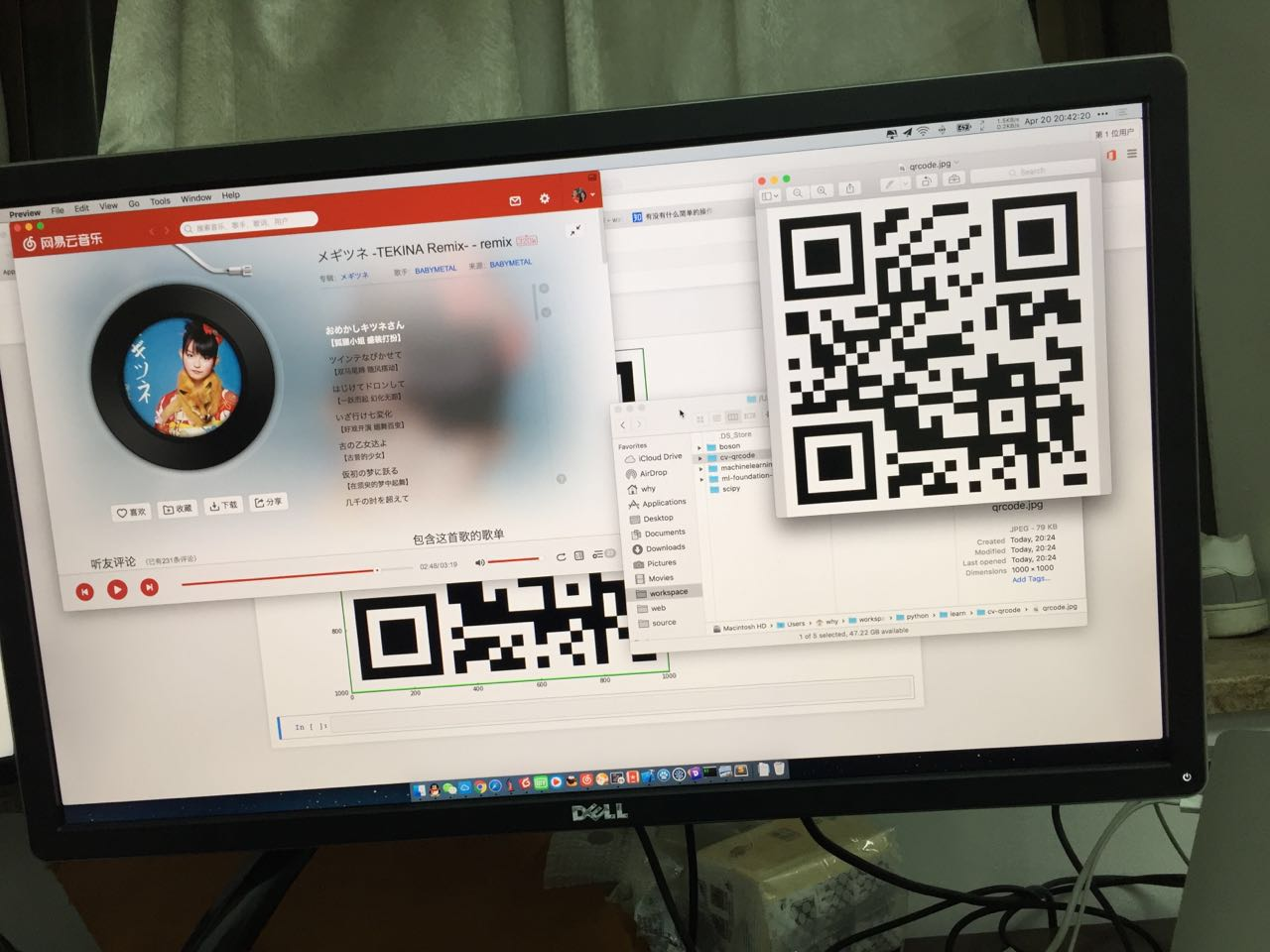### 加载图像

``````%matplotlib inline
import cv2
from matplotlib import pyplot as plt
import numpy as np

def show(img, code=cv2.COLOR_BGR2RGB):
cv_rgb = cv2.cvtColor(img, code)
fig, ax = plt.subplots(figsize=(16, 10))
ax.imshow(cv_rgb)
fig.show()

show(img)
``````

OpenCV 中默认是 BGR 通道，通过 cvtColor 函数将原图转换成灰度图：

``````img_gray = cv2.cvtColor(img, cv2.COLOR_BGR2GRAY)
``````

### 边缘检测

Canny 边缘检测算法主要是以下几个步骤：

• 用高斯滤波器平滑图像去除噪声干扰（低通滤波器消除高频噪声）。
• 通过非极大值抑制（non-maximum suppression）缩小边缘宽度。非极大值抑制的意思是，只保留梯度方向上的极大值，删除其他非极大值，从而实现锐化的效果。
• 通过双阈值法（double threshold）寻找潜在边缘。大于高阈值为强边缘（strong edge），保留；小于低阈值则删除；不大不小的为弱边缘（weak edge），待定。
• 通过迟滞现象（Hysteresis）处理待定边缘。弱边缘有可能是边缘，也可能是噪音，判断标准是：如果一个弱边缘点附近的八个相邻点中，存在一个强边缘，则此弱边缘为强边缘，否则排除。

``````img_gb = cv2.GaussianBlur(img_gray, (5, 5), 0)
``````

``````edges = cv2.Canny(img_gray, 100 , 200)
``````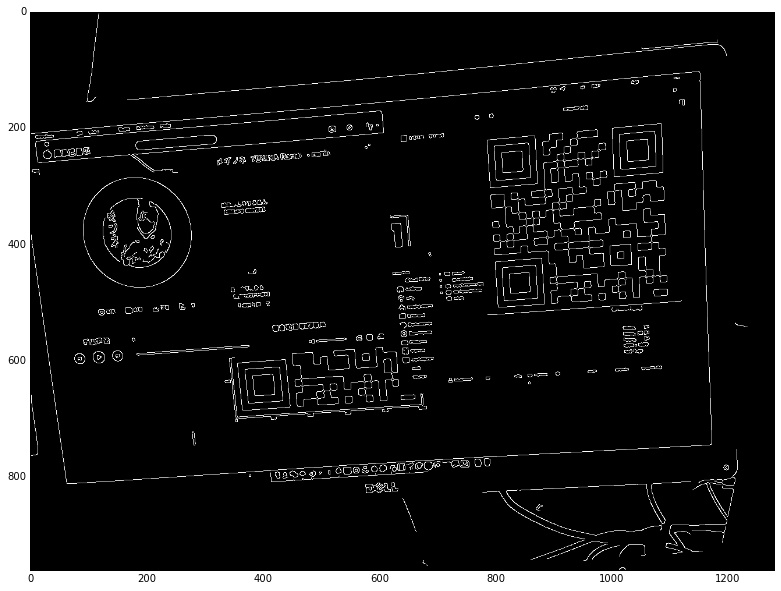### 寻找定位标记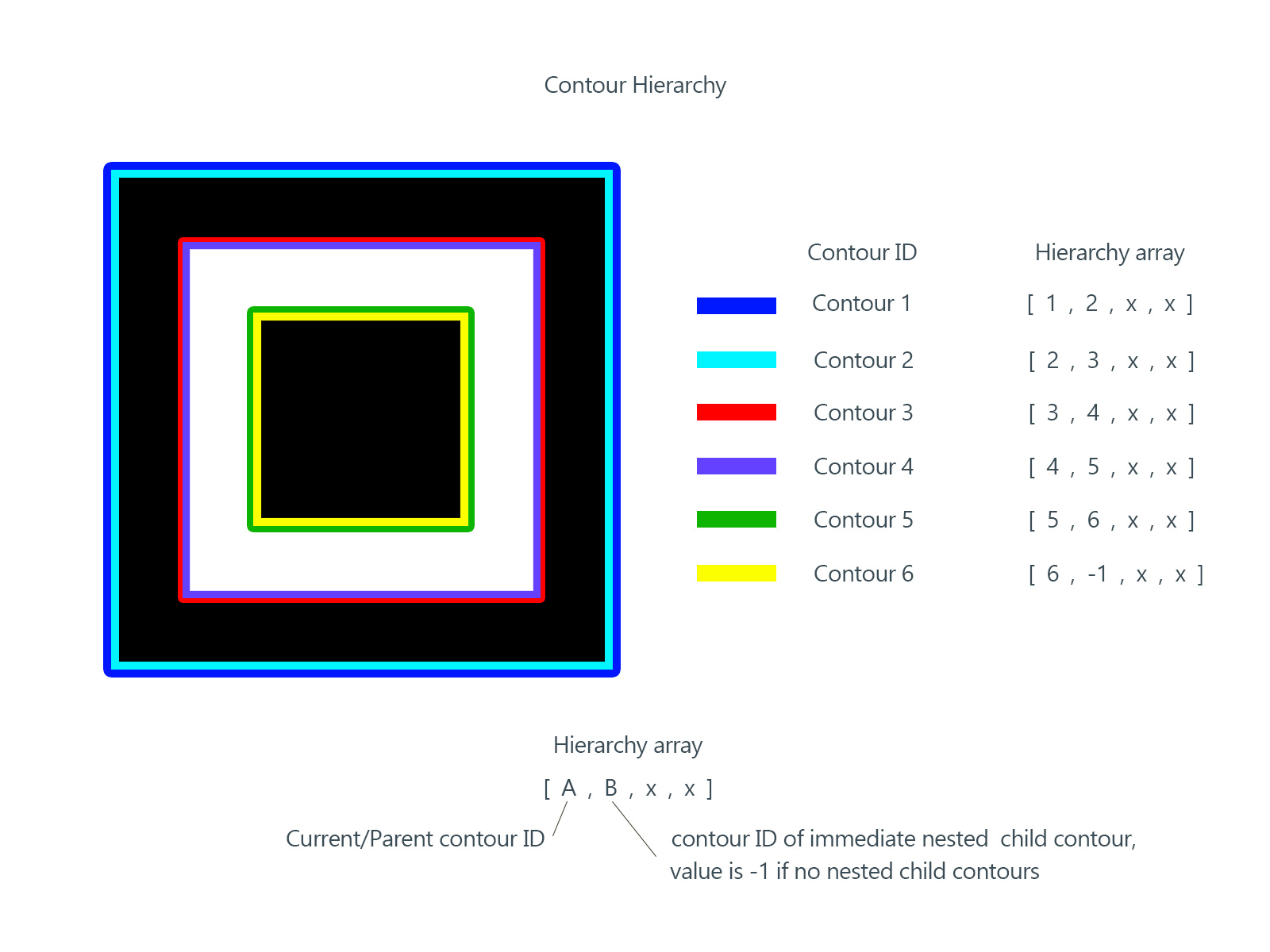``````img_fc, contours, hierarchy = cv2.findContours(edges, cv2.RETR_TREE, cv2.CHAIN_APPROX_SIMPLE)

hierarchy = hierarchy
found = []
for i in range(len(contours)):
k = i
c = 0
while hierarchy[k] != -1:
k = hierarchy[k]
c = c + 1
if c >= 5:
found.append(i)

for i in found:
img_dc = img.copy()
cv2.drawContours(img_dc, contours, i, (0, 255, 0), 3)
show(img_dc)
``````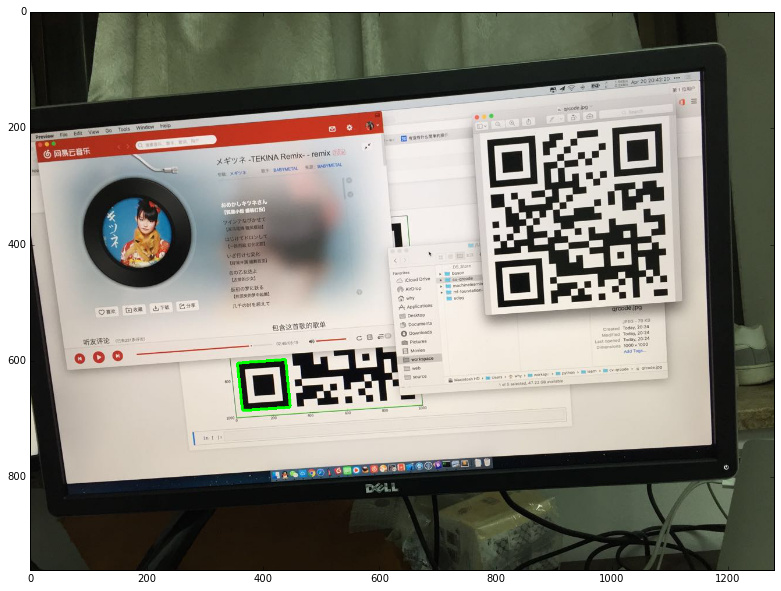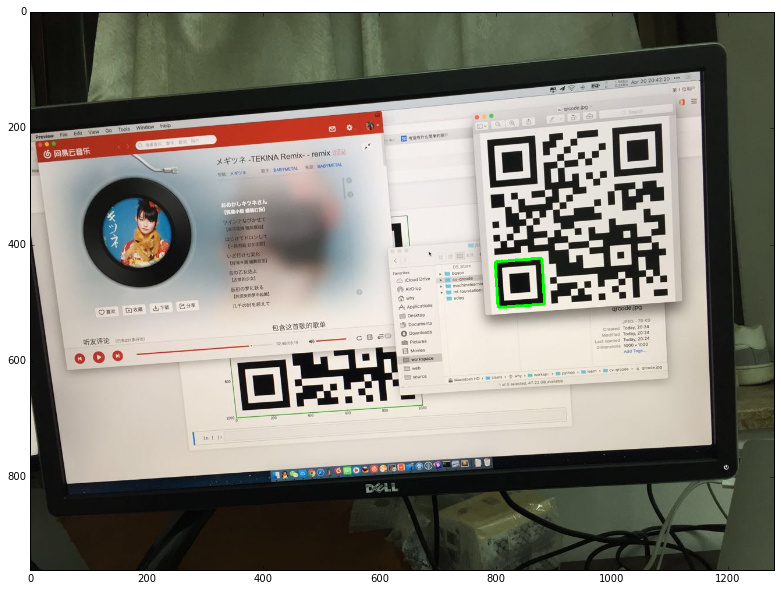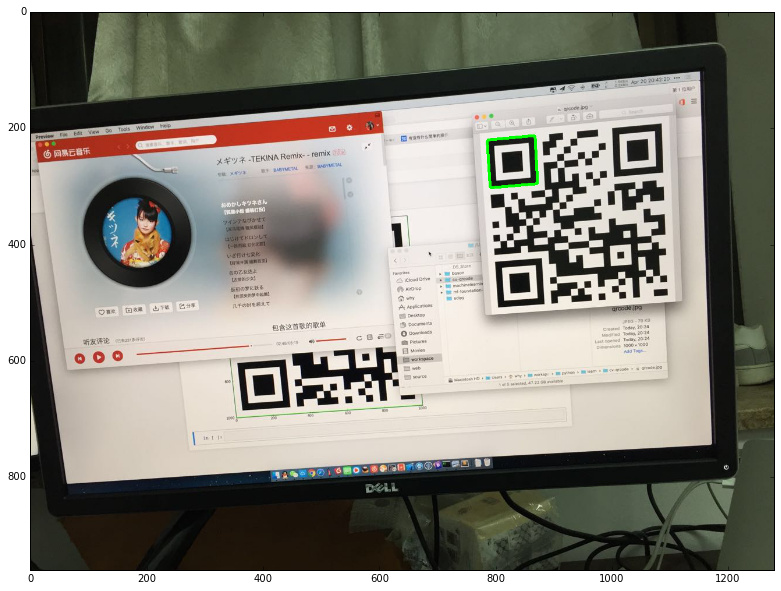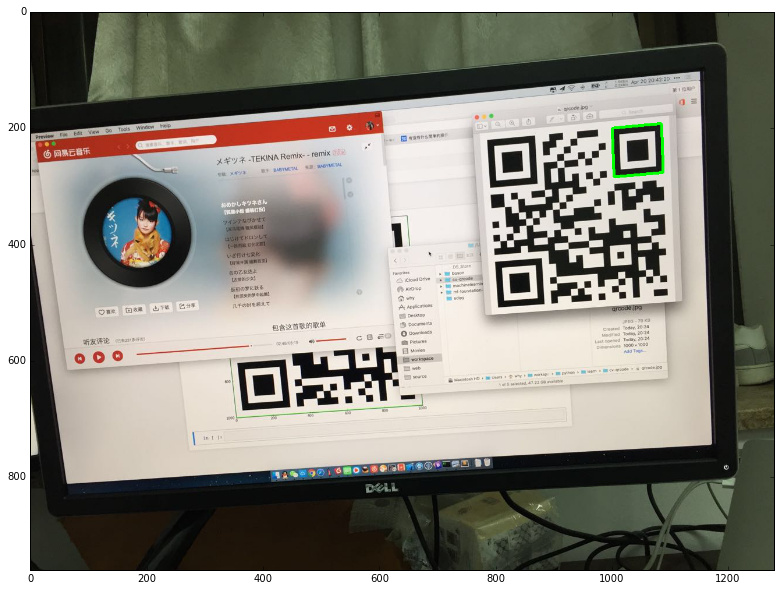### 定位筛选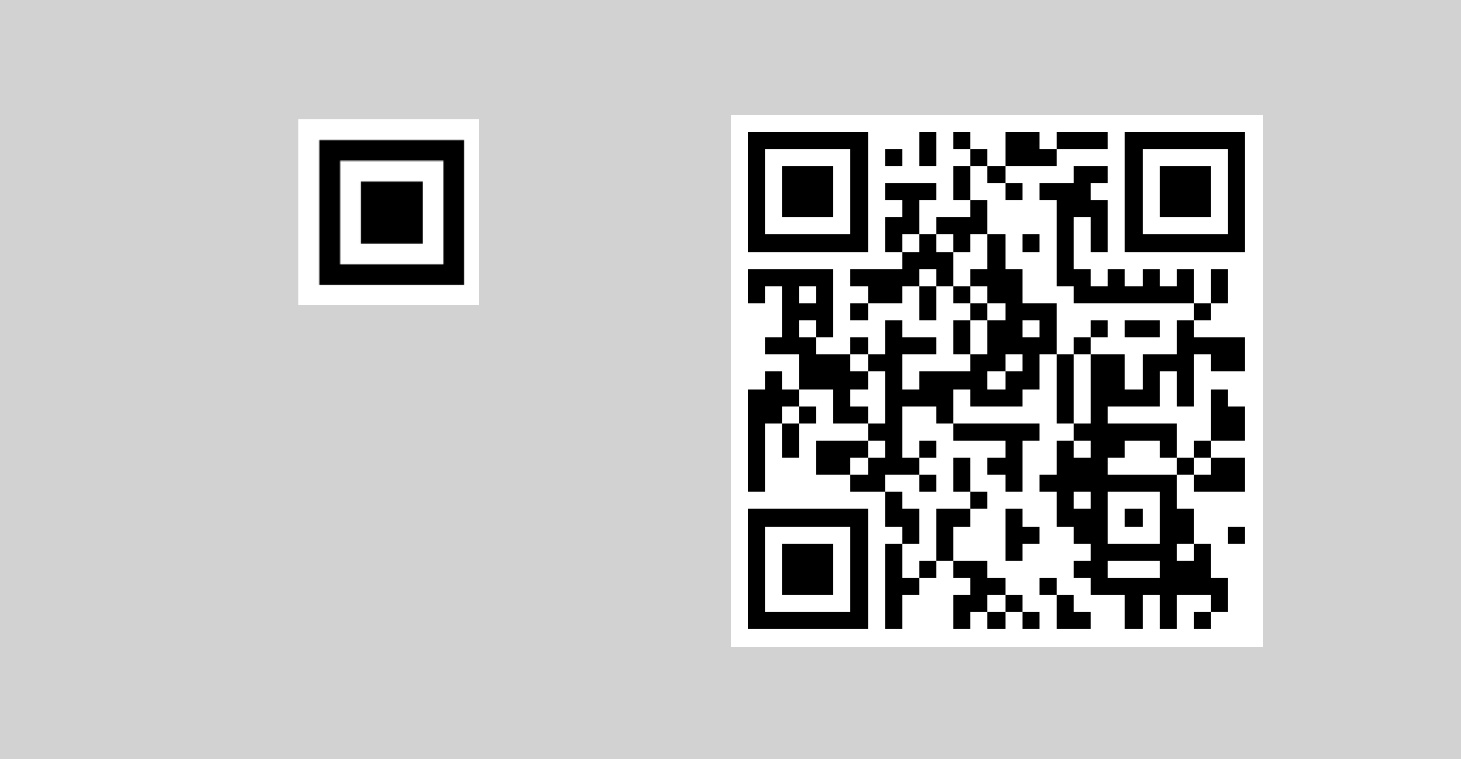• 将4个定位标记两两配对
• 将他们的4个顶点两两连线，选出最短的那两根
• 如果两根线都不符合 Timing Pattern 的特征，则出局

``````draw_img = img.copy()
for i in found:
rect = cv2.minAreaRect(contours[i])
box = cv2.boxPoints(rect)
box = np.int0(box)
cv2.drawContours(draw_img,[box], 0, (0,0,255), 2)
show(draw_img)
``````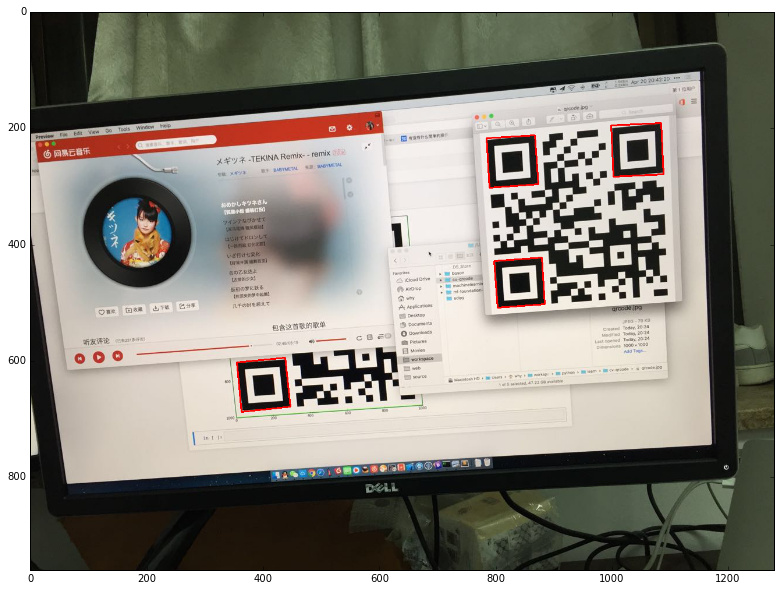``````boxes = []
for i in found:
rect = cv2.minAreaRect(contours[i])
box = cv2.boxPoints(rect)
box = np.int0(box)
box = map(tuple, box)
boxes.append(box)
``````

``````def cv_distance(P, Q):
return int(math.sqrt(pow((P - Q), 2) + pow((P - Q),2)))

def check(a, b):
# 存储 ab 数组里最短的两点的组合
s1_ab = ()
s2_ab = ()
# 存储 ab 数组里最短的两点的距离，用于比较
s1 = np.iinfo('i').max
s2 = s1
for ai in a:
for bi in b:
d = cv_distance(ai, bi)
if d < s2:
if d < s1:
s1_ab, s2_ab = (ai, bi), s1_ab
s1, s2 = d, s1
else:
s2_ab = (ai, bi)
s2 = d
a1, a2 = s1_ab, s2_ab
b1, b2 = s1_ab, s2_ab
# 将最短的两个线画出来
cv2.line(draw_img, a1, b1, (0,0,255), 3)
cv2.line(draw_img, a2, b2, (0,0,255), 3)

for i in range(len(boxes)):
for j in range(i+1, len(boxes)):
check(boxes[i], boxes[j])
show(draw_img)
``````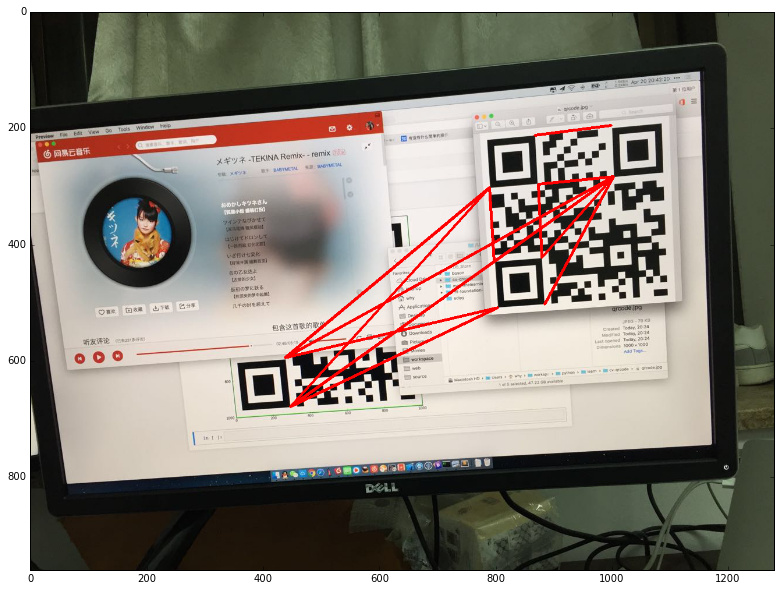``````th, bi_img = cv2.threshold(img_gray, 100, 255, cv2.THRESH_BINARY)
``````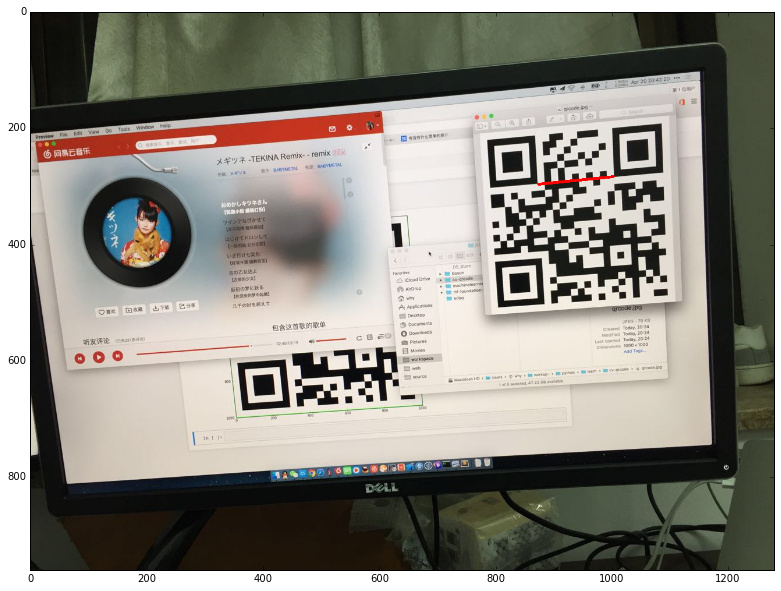``````[ 255.  255.  255.  255.  255.  255.  255.  255.  255.  255.  255.    0.
0.    0.    0.    0.    0.    0.    0.    0.    0.    0.    0.    0.
0.    0.    0.    0.    0.    0.    0.    0.    0.    0.    0.    0.
0.    0.    0.    0.    0.    0.    0.    0.    0.    0.    0.    0.
0.  255.  255.  255.    0.    0.    0.    0.    0.    0.    0.    0.
0.    0.    0.    0.    0.    0.    0.    0.    0.  255.  255.  255.
255.  255.  255.  255.  255.  255.  255.  255.  255.  255.  255.  255.
255.  255.  255.  255.  255.  255.  255.  255.  255.  255.  255.  255.
0.    0.    0.  255.  255.  255.  255.  255.  255.  255.  255.  255.
255.    0.  255.  255.  255.  255.  255.  255.  255.  255.  255.  255.
255.  255.  255.  255.  255.  255.  255.  255.  255.]
``````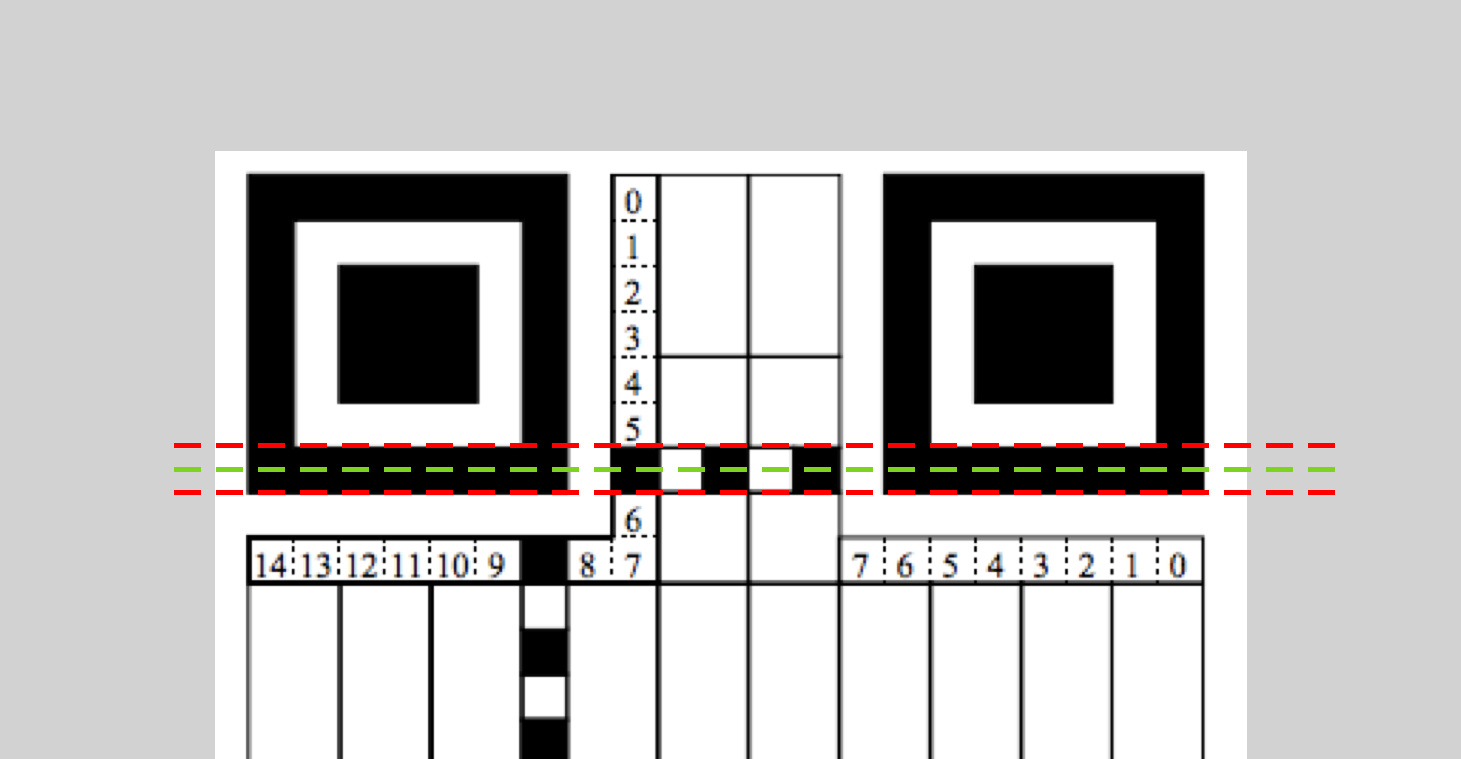``````a1 = (a1 + (a2-a1)*1/14, a1 + (a2-a1)*1/14)
b1 = (b1 + (b2-b1)*1/14, b1 + (b2-b1)*1/14)
a2 = (a2 + (a1-a2)*1/14, a2 + (a1-a2)*1/14)
b2 = (b2 + (b1-b2)*1/14, b2 + (b1-b2)*1/14)
``````

``````[ 255.  255.  255.  255.  255.  255.  255.  255.  255.  255.  255.    0.
0.    0.    0.    0.    0.    0.    0.    0.    0.    0.  255.  255.
255.  255.  255.  255.  255.  255.  255.  255.  255.  255.  255.    0.
0.    0.    0.    0.    0.    0.    0.    0.    0.    0.  255.  255.
255.  255.  255.  255.  255.  255.  255.  255.  255.  255.  255.    0.
0.    0.    0.    0.    0.    0.    0.    0.    0.    0.  255.  255.
255.  255.  255.  255.  255.  255.  255.  255.  255.  255.    0.    0.
0.    0.    0.    0.    0.    0.    0.    0.    0.    0.  255.  255.
255.  255.  255.  255.  255.  255.  255.  255.  255.  255.    0.    0.
0.    0.    0.    0.    0.    0.    0.    0.    0.    0.  255.  255.
255.  255.  255.  255.  255.  255.  255.  255.  255.]
``````

• 先除去数组中开头和结尾处连续的白色像素点。
• 对数组中的元素进行计数，相邻的元素如果值相同则合并到计数结果中。比如 [0,1,1,1,0,0] 的计数结果就是 [1,3,2] 。
• 计数数组的长度如果小于 5 ，则不是 Timing Pattern 。
• 计算计数数组的方差，看看分布是否离散，如果方差大于阈值，则不是 Timing Pattern 。

``````def isTimingPattern(line):
# 除去开头结尾的白色像素点
while line != 0:
line = line[1:]
while line[-1] != 0:
line = line[:-1]
# 计数连续的黑白像素点
c = []
count = 1
l = line
for p in line[1:]:
if p == l:
count = count + 1
else:
c.append(count)
count = 1
l = p
c.append(count)
# 如果黑白间隔太少，直接排除
if len(c) < 5:
return False
# 计算方差，根据离散程度判断是否是 Timing Pattern
threshold = 5
return np.var(c) < threshold
``````

``````[11, 12, 11, 12, 11, 12, 11, 13, 11]
``````

``````valid = set()
for i in range(len(boxes)):
for j in range(i+1, len(boxes)):
if check(boxes[i], boxes[j]):
print valid
``````

``````set([1, 2, 3])
``````

### 找出二维码

``````contour_all = np.array([])
while len(valid) > 0:
c = found[valid.pop()]
for sublist in c:
for p in sublist:
contour_all.append(p)

rect = cv2.minAreaRect(contour_ALL)
box = cv2.boxPoints(rect)
box = np.array(box)

draw_img = img.copy()
cv2.polylines(draw_img, np.int32([box]), True, (0, 0, 255), 10)
show(draw_img)
``````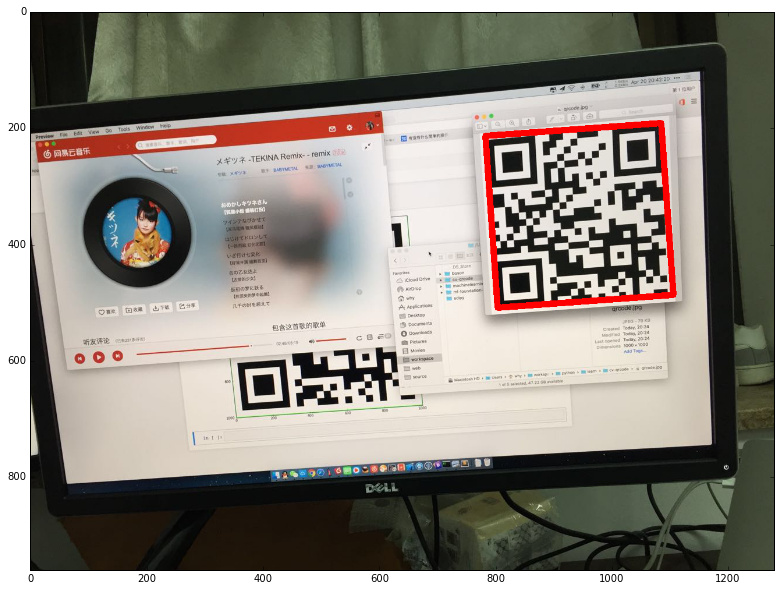## 小结

『费了半天劲，只是为了告诉我第0个定位标记是无效的，我看图也看出来了啊！』

+ 关注